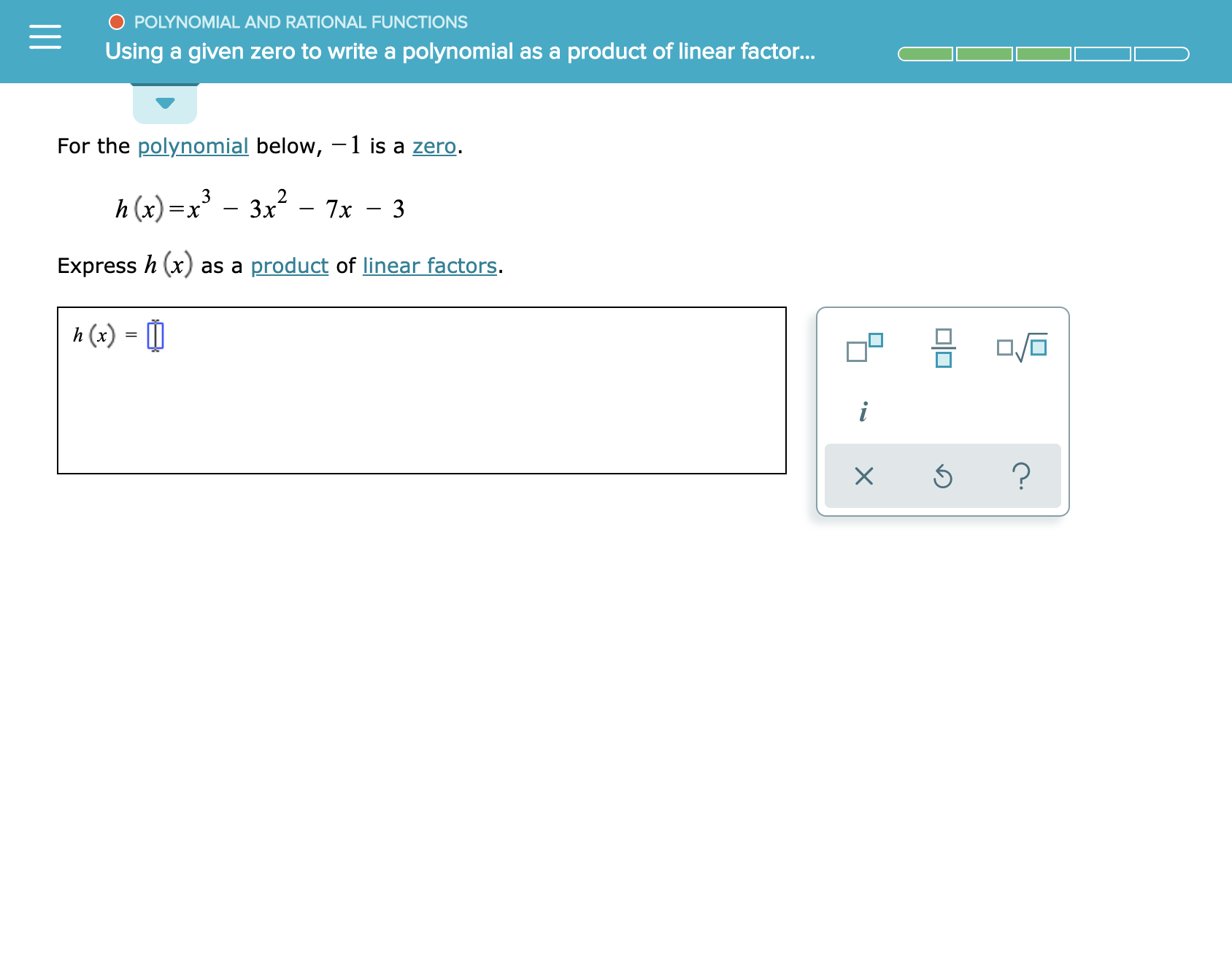# O POLYNOMIAL AND RATIONAL FUNCTIONSUsing a given zero to write a polynomial as a product of linear factor...For the polynomial below, 1 is a zero.3=X3x2-7x 3h(x)Express h (xas a product of linear factors.h (x)i?X

Question
52 views

See attachmenthelp_outlineImage TranscriptioncloseO POLYNOMIAL AND RATIONAL FUNCTIONS Using a given zero to write a polynomial as a product of linear factor... For the polynomial below, 1 is a zero. 3 =X 3x2-7x 3 h(x) Express h (x as a product of linear factors. h (x) i ? X fullscreen
check_circle

Step 1

Calculation:

Express the polynomial h(x) = x3 – 3x2 − 7x – 3 as the linear factors as follows.

...

### Want to see the full answer?

See Solution

#### Want to see this answer and more?

Solutions are written by subject experts who are available 24/7. Questions are typically answered within 1 hour.*

See Solution
*Response times may vary by subject and question.
Tagged in

### Calculus